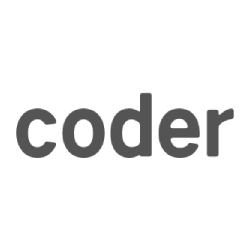# HDOJ4496（并查集，反向求解）

D-City

Time Limit: 2000/1000 MS (Java/Others) Memory Limit: 65535/65535 K (Java/Others)

Total Submission(s): 5582 Accepted Submission(s): 1957

Problem Description

Luxer is a really bad guy. He destroys everything he met.

One day Luxer went to D-city. D-city has N D-points and M D-lines. Each D-line connects exactly two D-points. Luxer will destroy all the D-lines. The mayor of D-city wants to know how many connected blocks of D-city left after Luxer destroying the first K D-lines in the input.

Two points are in the same connected blocks if and only if they connect to each other directly or indirectly.

Input

First line of the input contains two integers N and M.

Then following M lines each containing 2 space-separated integers u and v, which denotes an D-line.

Constraints:

0 < N <= 10000

0 < M <= 100000

0 <= u, v < N.

Output

Output M lines, the ith line is the answer after deleting the first i edges in the input.

Sample Input

5 10

0 1

1 2

1 3

1 4

0 2

2 3

0 4

0 3

3 4

2 4

Sample Output

1

1

1

2

2

2

2

3

4

5

Hint

The graph given in sample input is a complete graph, that each pair of vertex has an edge connecting them, so there’s only 1 connected block at first.

The first 3 lines of output are 1s because after deleting the first 3 edges of the graph, all vertexes still connected together.

But after deleting the first 4 edges of the graph, vertex 1 will be disconnected with other vertex, and it became an independent connected block.

Continue deleting edges the disconnected blocks increased and finally it will became the number of vertex, so the last output should always be N.

``````//拆除线路判断是否断开不好判断，
//所以反向连接判断是否形成区域
#include<iostream>
#include<algorithm>
#include<stdio.h>
#include<string.h>
#include<stdlib.h>
#include<queue>
#define MAXN 10005
using namespace std;

int operation [][];//记录指令
int father[MAXN];
int sum[];//记录结果
int num;
int n,m;

//并查集基本操作
int mfind(int x){
int r = x;
while(r!=father[r]){
r = father[r];
}
int i=x,j;
while(i!=r){
j = father[i];
father[i] = r;
i = j;
}
return r;
}
void connect(int x,int y){
int nx = mfind(x);
int ny = mfind(y);
if(nx!=ny){
father[ny] = nx;
num--;
}
}

int main()
{
while(~scanf("%d%d",&n,&m)){
num = n;
for(int i=;i<=n;i++){
father[i] = i;
}
for(int i=;i<m;i++){
scanf("%d%d",&operation[i][],&operation[i][]);
}
for(int i=m-;i>=;i--){
sum[i] = num;
connect(operation[i][],operation[i][]);
}
for(int i=;i<m;i++){
printf("%d\n",sum[i]);
}
}
return ;
}
``````